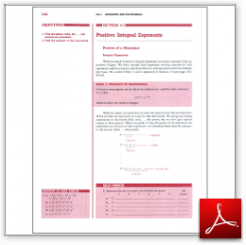Algebra Math Book - Introductory Algebra Thank you for your continual support.

 The power of mathematics rests in the logic of thinking.ID: Sec4-1
Description: Powers of a Monomial-Raising a Power to a Power-Product to a Power-Quotient to a Power
Price: 1.99

# Algebra Math book - Introductory Algebra - Chapter 4 - Section 1 - Positive Integral Exponents

## Section 4.1 - Objectives

1.  Find the square, cube, 4th,...,nth powers of a monomial.

2.  Find the quotient of two monomials.

Navigate to
Next Section
or Chapter 4 Details or Other Chapters

This section of my algebra math book, Introductory Algebra, also includes in the download:

• Cover Sheet